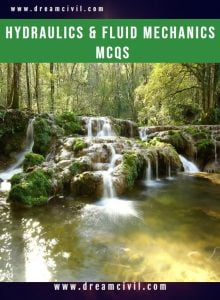Wednesday, October 4, 2023

Hydraulics & Fluid Mechanics Objective Questions ( MCQs ) PDF containing 515 Hydraulics & 60 Fluid questions is given below. You can directly download this PDF and study from it.

### 1. Details of the PDFTopic of MCQs Hydraulics & Fluid Mechanics Size 919 KB Pages 103 Number of Questions 515 Hydraulics & 60 Fluids

### 2. Some questions from PDF

Question No. 1
The error in discharge (dQ/Q) to the error in measurement of the head (dH/H) over a rectangular notch is given by

(A) dQ/Q = (1/2) × (dH/H)
(B) dQ/Q = (3/4) × (dH/H)
(C) dQ/Q = (dH/H)
(D) dQ/Q = (3/2) × (dH/H)

Question No. 2
The flow of water through the hole in the bottom of a washbasin is an example of

(B) Uniform flow
(C) Free vortex
(D) Forced vortex

Question No. 3
A flow in which the viscosity of the fluid is dominating over the inertia force is called

(C) Laminar flow
(D) Turbulent flow

Question No. 4
The hydraulic mean depth or the hydraulic radius is the ratio of

(A) Area of flow and wetted perimeter
(B) Wetted perimeter and diameter of the pipe
(C) Velocity of flow and area of flow
(D) None of these

Question No. 5
A flow whose streamline is represented by a curve is called

(A) One-dimensional flow
(B) Two-dimensional flow
(C) Three-dimensional flow
(D) Four-dimensional flow

Question No. 6
The value of the coefficient of discharge is __________ the value of the coefficient of velocity.

(A) Less than
(B) Same as
(C) More than
(D) None of these

Question No. 7
The hydraulic mean depth for a circular pipe of diameter (d) is

(A) d/6
(B) d/4
(C) d/2
(D) d

Question No. 8
A fluid whose viscosity does not change with the rate of deformation or shear strain is known as

(A) Real fluid
(B) Ideal fluid
(C) Newtonian fluid
(D) Non-Newtonian fluid

Question No. 9
The barometer is used to measure

(A) Velocity of liquid
(B) Atmospheric pressure
(C) Pressure in pipes and channels
(D) Difference of pressure between two points in a pipe

Question No. 10
An error of 1% in measuring head over the crest of the notch (H) will produce an error of __________ in discharge over a triangular notch,

(A) 1 %
(B) 1.5 %
(C) 2 %
(D) 2.5 %

Question No. 11
The coefficient of venturi-flume, generally, lies between

(A) 0.3 to 0.45
(B) 0.50 to 0.75
(C) 0.75 to 0.95
(D) 0.95 to 1.0

Question No. 12
A flow in which the quantity of liquid flowing per second is not constant is called

(A) Streamline flow
(B) Turbulent flow

Question No. 13
The specific gravity of water is taken as

(A) 0.001
(B) 0.01
(C) 0.1
(D) 1

Thank you ! for visiting us. If you have any queries then kindly mail us at madhukrishnapoudel151@gmail.com or WhatsApp us at 9804406747.He is a founder and lead author of Dream Civil International and his civil engineering research articles has been taken as source by world's top news and educational sites like USA Today, Time, The richest, Wikipedia, etc.
Latest Articles

Related Articles